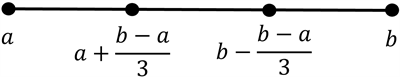# Cantor集与Cantor函数性质探究Research on the Properties of Cantor Sets and Cantor Functions

DOI: 10.12677/AAM.2021.104132, PDF, HTML, XML, 下载: 9  浏览: 27

Abstract: The Cantor set was introduced by the German mathematician Georg Cantor in 1883. Because of its ingenious conception and unique nature, Cantor set is widely used and provides ideas and methods for solving many mathematical problems. Cantor function is constructed from Cantor set, and its special properties can also be applied to many mathematical problems. Their special and wonderful nature makes them incomparable charm, which attracts many mathematicians to explore and study them. Starting from the construction of Cantor set and Cantor function, this paper mainly discusses the properties of Cantor set and Cantor function.

1. 引言

Cantor集是由德国数学家格奥尔格·康托尔在1883年引入的。因其构思精巧且性质独特，Cantor集应用广泛并且为众多数学问题提供了解决的思路和方法。而且，康托尔和其他数学家通过考虑Cantor集奠定了现代点集拓扑学的基础。而Cantor函数是定义在 $\left[0,1\right]$ 上的一个连续的、单调递增的、几乎处处可微的函数，在实际问题的求解中扮演着重要的角色，而且Cantor函数特殊奇妙的性质使得其获得无与伦比的魅力，吸引了众多数学工作者对它进行探索和研究。如它不满足Newton-Leibniz公式，它可以将疏朗集映成连续区间，它在 $\left[0,1\right]$ 上的导数 ${\Theta }^{\prime }\left(x\right)$ 几乎处处等于0，即 ${\Theta }^{\prime }\left(x\right)=0$ a.e.于 $\left[0,1\right]$${\int }_{\left[0,1\right]}{\Theta }^{\prime }\left(x\right)\text{d}m=0$ 等。如此多特殊且奇妙的性质使得Cantor函数有着无与伦比的魅力吸引了众多数学工作者对它进行探索和研究。

2. Cantor集

2.1. Cantor集构造

Cantor集是数学领域中一个十分重要的点集，也称为Cantor三分集或者Cantor完全集。有界闭区间 $\left[a,b\right]\left(a 上的Cantor集的构造思想如下：Figure 1. Schematic diagram of trisection of bounded closed interval $\left[a,b\right]$

${F}^{1}=F\left(\left[a,b\right]\right)=\left[a,a+\frac{b-a}{3}\right]\cup \left[b-\frac{b-a}{3},b\right];$

$\begin{array}{l}{F}^{2}=F\left(F\left(\left[a,b\right]\right)\right)=\left[a,a+\frac{b-a}{{3}^{2}}\right]\cup \left[a+\frac{2\left(b-a\right)}{{3}^{2}},a+\frac{3\left(b-a\right)}{{3}^{2}}\right]\\ \text{\hspace{0.17em}}\text{\hspace{0.17em}}\text{\hspace{0.17em}}\text{\hspace{0.17em}}\text{\hspace{0.17em}}\text{\hspace{0.17em}}\text{\hspace{0.17em}}\text{\hspace{0.17em}}\text{\hspace{0.17em}}\text{\hspace{0.17em}}\text{\hspace{0.17em}}\text{\hspace{0.17em}}\text{\hspace{0.17em}}\text{\hspace{0.17em}}\text{\hspace{0.17em}}\text{\hspace{0.17em}}\text{\hspace{0.17em}}\text{\hspace{0.17em}}\text{\hspace{0.17em}}\text{\hspace{0.17em}}\text{\hspace{0.17em}}\text{\hspace{0.17em}}\text{\hspace{0.17em}}\text{\hspace{0.17em}}\text{\hspace{0.17em}}\text{\hspace{0.17em}}\text{\hspace{0.17em}}\text{\hspace{0.17em}}\cup \left[b-\frac{3\left(b-a\right)}{{3}^{2}},b-\frac{2\left(b-a\right)}{{3}^{2}}\right]\cup \left[b-\frac{b-a}{{3}^{2}},b\right].\end{array}$

$\begin{array}{c}{F}^{k}=F\left({F}^{k-1}\left(\left[a,b\right]\right)\right)\\ =\left[a,a+\frac{b-a}{{3}^{k}}\right]\cup \left[a+\frac{2\left(b-a\right)}{{3}^{k}},a+\frac{3\left(b-a\right)}{{3}^{k}}\right]\cup \cdots \\ \text{\hspace{0.17em}}\cup \left[b-\frac{\left({3}^{k}-3\right)\left(b-a\right)}{{3}^{k}},b-\frac{\left({3}^{k}-2\right)\left(b-a\right)}{{3}^{k}}\right]\cup \left[b-\frac{\left({3}^{k}-1\right)\left(b-a\right)}{{3}^{k}},b\right].\end{array}$

$C=\underset{k\to \infty }{\mathrm{lim}}{F}^{k}\left(\left[a,b\right]\right).$

$G\triangleq \left(\left[0,1\right]\C\right)$ 为Cantor余集。

2.2. Cantor集性质及证明

$\phi \left(t\right)=\left(1-t\right)a+tb,t\in \left[0,1\right]$

$\forall {y}_{0}\in \left({x}_{0}-\delta ,{x}_{0}+\delta \right)\cap G$ 。因为 ${y}_{0}$ 是开集 $\left({x}_{0}-\delta ,{x}_{0}+\delta \right)\cap G$ 中的点，故存在 $\epsilon >0$ ，使得 $\cup \left({y}_{0},\epsilon \right)\subset \left({x}_{0}-\delta ,{x}_{0}+\delta \right)\cap G$ ，亦即 $\cup \left({y}_{0},\epsilon \right)\subset \left({x}_{0}-\delta ,{x}_{0}+\delta \right)$$\cup \left({y}_{0},\epsilon \right)\cap C=\varnothing$ 。由 ${y}_{0}$ 的任意性知C的内部是空集，即C是疏朗集。

$G=\underset{i=1}{\overset{\infty }{\cup }}\left(\left[0,1\right]\{F}^{i}\left(\left[0,1\right]\right)\right)$

$\underset{i=1}{\overset{\infty }{\sum }}\frac{{2}^{i-1}}{{3}^{i}}=1.$

$C=\underset{i=1}{\overset{\infty }{\cap }}{F}^{i},$

${m}^{*}\left(C\right)\le {m}^{*}\left({F}^{i}\right)\le {2}^{i}\cdot {3}^{-i},$

$\left[0,1\right]$ 中的点用三进制小数表示，则对 $\left[0,1\right]$ 中任意一点 $x\in \left[0,1\right]$$x=0.{a}_{1}{a}_{2}\cdots {a}_{k}\cdots =\frac{{a}_{1}}{3}+\frac{{a}_{2}}{{3}^{2}}+\cdots +\frac{{a}_{k}}{{3}^{k}}+\cdots$ ，其中 ${a}_{i}=0,1,2\left(i=1,2,\cdots ,k,\cdots \right)$

$\forall {x}_{0}\in \left[0,1\right]\cap C$ 。因 ${x}_{0}$ 为C中的点，由Cantor集的构造过程可知，将 ${x}_{0}$ 用上述三进制小数表示成 ${x}_{0}=0.{b}_{1}{b}_{2}\cdots {b}_{k}\cdots$ 具有特点 ${b}_{i}=0,2\left(i=1,2,\cdots ,k,\cdots \right)$

3. Cantor函数

3.1. Cantor函数构造

$\left(\frac{1}{{3}^{k}},\frac{2}{{3}^{k}}\right),\left(\frac{7}{{3}^{k}},\frac{8}{{3}^{k}}\right),\left(\frac{19}{{3}^{k}},\frac{20}{{3}^{k}}\right),\cdots ,\left(\frac{{3}^{k}-2}{{3}^{k}},\frac{{3}^{k}-1}{{3}^{k}}\right)$

$\frac{1}{{2}^{k}},\frac{3}{{2}^{k}},\frac{5}{{2}^{k}},\cdots ,\frac{{2}^{k}-1}{{2}^{k}}.$

$x\in C,x\notin \left\{0,1\right\}$ 时，定义

$\Theta \left(x\right)=\mathrm{sup}\left\{\Theta \left(t\right)|t\in G,t

$\Theta \left(x\right)=\left\{\begin{array}{ll}0\hfill & x=0\hfill \\ 1\hfill & x=1\hfill \\ \frac{1}{2}\hfill & x\in \left(\frac{1}{3},\frac{2}{3}\right)\hfill \\ \frac{1}{{2}^{2}}\hfill & x\in \left(\frac{1}{{3}^{2}},\frac{2}{{3}^{2}}\right)\hfill \\ \frac{3}{{2}^{2}}\hfill & x\in \left(\frac{7}{{3}^{2}},\frac{8}{{3}^{2}}\right)\hfill \\ \text{\hspace{0.17em}}⋮\hfill & \text{\hspace{0.17em}}\text{\hspace{0.17em}}\text{\hspace{0.17em}}\text{\hspace{0.17em}}\text{\hspace{0.17em}}\text{\hspace{0.17em}}\text{\hspace{0.17em}}\text{\hspace{0.17em}}\text{\hspace{0.17em}}⋮\hfill \\ \frac{1}{{2}^{k}}\hfill & x\in \left(\frac{1}{{3}^{k}},\frac{2}{{3}^{k}}\right)\hfill \\ \frac{3}{{2}^{k}}\hfill & x\in \left(\frac{7}{{3}^{k}},\frac{8}{{3}^{k}}\right)\hfill \\ \frac{5}{{2}^{k}}\hfill & x\in \left(\frac{19}{{3}^{k}},\frac{20}{{3}^{k}}\right)\hfill \\ \text{\hspace{0.17em}}⋮\hfill & \text{\hspace{0.17em}}\text{\hspace{0.17em}}\text{\hspace{0.17em}}\text{\hspace{0.17em}}\text{\hspace{0.17em}}\text{\hspace{0.17em}}\text{\hspace{0.17em}}\text{\hspace{0.17em}}\text{\hspace{0.17em}}⋮\hfill \\ \frac{{2}^{k}-1}{{2}^{k}}\hfill & x\in \left(\frac{{3}^{k}-2}{{3}^{k}},\frac{{3}^{k}-1}{{3}^{k}}\right)\hfill \\ \text{\hspace{0.17em}}⋮\hfill & \text{\hspace{0.17em}}\text{\hspace{0.17em}}\text{\hspace{0.17em}}\text{\hspace{0.17em}}\text{\hspace{0.17em}}\text{\hspace{0.17em}}\text{\hspace{0.17em}}\text{\hspace{0.17em}}\text{\hspace{0.17em}}⋮\hfill \\ \mathrm{sup}\left\{\Theta \left(t\right)|t\in G,t

3.2. Cantor函数性质及证明

$\left[{x}_{1},{y}_{1}\right],\left[{x}_{2},{y}_{2}\right],\cdots ,\left[{x}_{p},{y}_{p}\right],$

$p={2}^{k},$

$0={x}_{1}<{y}_{1}\le {x}_{2}<{y}_{2}\le \cdots \le {x}_{p}<{y}_{p}=1,$

${y}_{i}-{x}_{i}=\frac{1}{{3}^{k}},\text{\hspace{0.17em}}\text{\hspace{0.17em}}i=1,2,\cdots ,p.$

$\underset{i=1}{\overset{k}{\sum }}|{y}_{i}-{x}_{i}|=\frac{p}{{3}^{k}}=\frac{{2}^{k}}{{3}^{k}}<\delta .$

$0=\text{Θ}\left({x}_{1}\right)<\text{Θ}\left({y}_{1}\right)=\text{Θ}\left({x}_{2}\right)<\text{Θ}\left({y}_{2}\right)=\cdots =\text{Θ}\left({x}_{p}\right)<\text{Θ}\left({y}_{p}\right)=1,$

$\underset{i=1}{\overset{k}{\sum }}|\text{Θ}\left({y}_{i}\right)-\text{Θ}\left({x}_{i}\right)|=1>\frac{1}{2}={\epsilon }_{0}.$

4. Cantor集和Cantor函数的应用

Cantor集和Cantor函数的构思巧妙，性质十分特别。Cantor集是Cantor在解三角级数问题时做出来的，它具有若干重要特征。Cantor函数是由Cantor集构造的，它的特异性质也可以应用在很多数学问题中。

4.1. Cantor集可作为否定命题的反例

i) Lebesgue可测集一定是Borel集 。

Cantor集不为Borel集且它的外测度为零，故为Lebesgue可测集。

ii) 测度为零的集合一定是可列集 。

iii) 疏朗集均为孤立点集。

Cantor集为疏朗集，但 ${C}^{\prime }=C$ 是完全集，故不为孤立点集。

iv) 不含有孤立点的非空闭集一定含有内点。

Cantor是不含有孤立点的非空闭集，但由于其还是疏朗集，故其没有内点。

4.2. Cantor函数可作为否定命题的反例

i) 在连续映射下可测集的象一定可测。

Cantor函数 $\text{Θ}\left(x\right)$ ，令 $f\left(x\right)=\frac{x+\text{Θ}\left(x\right)}{2}$ ，则 $f:\left[0,1\right]\to \left[0,1\right]$ 为严格单调增的连续函数。并使得 $m\left(f\left(C\right)\right)=\frac{1}{2}$ ，其中C为Cantor集，取不可测集 $W\subset f\left(C\right)$ ，则有 ${f}^{-1}\left(W\right)\subset C$ 可测，使 $f\left({f}^{-1}\left(W\right)\right)=W$ 不可测。

ii) 在连续映射下可测集的原象一定可测。

iii) 所有连续函数均满足Newton-Leibniz公式。

Cantor函数 $\Theta \left(x\right)$ 不满足Newton-Leibniz公式。Cantor函数 $\Theta \left(x\right)$$\left[0,1\right]$ 上几乎处处可微，且 ${\Theta }^{\prime }\left(x\right)=0$ a.e.于 $\left[0,1\right]$ ，在 $\left[0,1\right]$ 上几乎处处连续，故R-可积。但是

${\int }_{0}^{1}{\Theta }^{\prime }\left(x\right)\text{d}x=0<1=\text{Θ}\left(1\right)-\text{Θ}\left(0\right).$

  董大校. Cantor集性质的应用[J]. 玉溪师范学院学报, 2009, 25(8): 18-22.  周民强. 实变函数论[M]. 第3版. 北京: 北京大学出版社, 2016.  李翠香, 石凌, 刘丽霞. Cantor集的性质及应用[J]. 大学数学, 2011, 27(2): 156-158.  吴杰, 夏雪. 著名的Cantor函数及其应用实例[J]. 高等函授学报(自然科学版), 2004, 18(5): 26-27.  黄珊. 对Cantor函数单调连续性的探究[J]. 新课程(教研版), 2012(5): 106-107.  王晶昕, 王炜, 任咏红. 实变函数论[M]. 北京: 科学出版社, 2006.